## research papersBIOLOGICALCRYSTALLOGRAPHY
ISSN: 1399-0047
Volume 63| Part 1| January 2007| Pages 101-107

## Ligand identification using electron-density map correlations

aLos Alamos National Laboratory, Mailstop M888, Los Alamos, NM 87545, USA, and bLawrence Berkeley National Laboratory, One Cyclotron Road, BLDG 64R0121, Berkeley, CA 94720, USA
*Correspondence e-mail: terwilliger@lanl.gov

(Received 26 January 2006; accepted 2 November 2006)

A procedure for the identification of ligands bound in crystal structures of macromolecules is described. Two characteristics of the density corresponding to a ligand are used in the identification procedure. One is the correlation of the ligand density with each of a set of test ligands after optimization of the fit of that ligand to the density. The other is the correlation of a fingerprint of the density with the fingerprint of model density for each possible ligand. The fingerprints consist of an ordered list of correlations of each the test ligands with the density. The two characteristics are scored using a Z-score approach in which the correlations are normalized to the mean and standard deviation of correlations found for a variety of mismatched ligand-density pairs, so that the Z scores are related to the probability of observing a particular value of the correlation by chance. The procedure was tested with a set of 200 of the most commonly found ligands in the Protein Data Bank, collectively representing 57% of all ligands in the Protein Data Bank. Using a combination of these two characteristics of ligand density, ranked lists of ligand identifications were made for representative (FoFc)exp(iφc) difference density from entries in the Protein Data Bank. In 48% of the 200 cases, the correct ligand was at the top of the ranked list of ligands. This approach may be useful in identification of unknown ligands in new macromolecular structures as well as in the identification of which ligands in a mixture have bound to a macromolecule.

### 1. Introduction

It is common in macromolecular crystal structure determinations to find that a small-molecule ligand has been cocrystallized with the macromolecule, even in cases where this ligand was not known to be present in the crystallization media. This situation occurs, for example, if the protein has been expressed in a complicated cell-based system containing many compounds and some of these compounds bind to the macromolecule and remain bound throughout purification and crystallization (see, for example, Zarembinski et al., 1998). The identification of the ligand in these cases can be an important step in characterizing the macromolecule, as it may give clues as to the natural function of the macromolecule. A related situation occurs in increasingly many drug-discovery and ligand-discovery projects in which a mixture of ligands is included in crystallization or after crystallization, a structure is determined and the identify of the bound ligand is determined from the density (see, for example, Tickle et al., 2004).

We have recently developed an approach to the fitting of flexible ligands to electron-density maps that is well suited to large-scale automated analyses (Terwilliger et al., 2006). The ligand-fitting approach is quite similar to the process that an expert crystallographer would follow; it consists of the identification of an optimal location and orientation of a core fragment of the ligand within the largest contiguous region of density in the map, followed by building the remainder of the ligand by tracing the density out from this core region. We previously have used this approach to build 9327 ligands from the Protein Data Bank (PDB; Berman et al., 2000) into (Fo − Fc)exp(iφc) difference density created by removal of ligands from entries from the PDB and found that 68% of these ligands could be rebuilt with an r.m.s.d. from the original coordinates of 2 Å or less. Several other methods for automatic fitting of ligand density have been developed recently (Diller et al., 1999; Oldfield, 2001; Zwart et al., 2004) and these could also most likely be used in the procedures we describe below.

Here, we evaluate the utilities of two approaches to ligand identification using electron density alone. The first approach is simply to fit each of a large set of possible ligands to the density and rank these ligands based on the correlation of calculated and observed density. The second approach extends this by creating a fingerprint' of correlations expected for density from each of a set of possible ligands and comparing this fingerprint with that obtained using the observed density in the map to identify the ligand. We test these approaches by applying them to examples of 200 of the most frequently found ligands from the PDB.

### 2. Methods

#### 2.1. Models, structure factors and ligands from the PDB

We began with 27 812 entries from the November 2004 release of the PDB stored in an Oracle database populated, using version 1.5.1 of the openMMS Toolkit (Greer et al., 2002), from mmCIF files obtained at ftp://beta.rcsb.org/pub/pdb/uniformity/data/mmCIF/divided . We selected the 12 001 entries that contained at least one large polypeptide molecule (20 or more residues) and one ligand, which we defined as a nonmacromolecular mmCIF entity with 6–150 non-H atoms and, if a polypeptide, containing no more than two residues. From these entries, we selected the 7025 entries that contained structure-factor amplitudes or intensities that, with minor automated editing, could be read by the CCP4 program cif2mtz (Collaborative Computational Project, Number 4, 1994). These 7025 entries contained 23 514 total instances of ligands, of which 22 562 (96%) could be successfully analyzed by our algorithms. The 22 562 ligands represent 2740 different ligand compounds, as defined by an ordered string of heterocompound codes (one for each residue in the mmCIF entity). The number of PDB entries containing each ligand was counted and the most common 200 were noted. These 200 most common ligands in our data set ranged from 658 PDB entries containing HEM (heme) and 593 with GOL (glycerol) to six entries with NAG-NAG-BMA. Some of these ligands had the same number and ordered list (by atom name) of non-H atoms in each instance, but many had some variability in the number and listing of non-H atoms, with some instances missing some or even the majority of atoms compared with another. For some purposes we further subdivided instances of each ligand into sets of instances in which both the heterocompound string and the list of non-H atom names were unique, calling these more exacting groupings the set of unique ligands', of which there were total of 3364 in the subset of the PDB we analyzed. The 200 unique ligands that were used in this study account for 57% of all ligands in the PDB. That is, 22 538 of the 39 607 ligand instances in the entire PDB match one of these 200 unique ligands both in heterocompound string and the list of non-H atoms.

We carried out the ligand-identification procedures as follows. For each of the most common 200 ligands from our data set, we chose one PDB entry that contained the ligand, along with that instance of the ligand, as an example. The examples were chosen arbitrarily (alphabetically) from a list of all entries that (i) contained the most complete version of this ligand (i.e. the most atoms) and (ii) had a correlation of (FoFc)exp(iφc) difference density calculated after removal of this ligand with model density calculated from the original ligand from the PDB entry of at least 0.75. If no entry satisfied the second condition, then the entry with the highest value of the correlation of density was chosen. A total of 200 FoFc difference density maps were obtained from the 200 ligand–PDB entry combinations by removal of the ligand followed by calculation of maps. The corresponding 200 ligands were each used to fit the 200 difference maps, except that in cases where a ligand was to be fitted to the PDB entry that it came from, a second example of that ligand (with the identical listing of non-H atoms) from a different PDB entry was used as a starting point for fitting. In this way, the original conformation could not be simply placed into density without any actual fitting of torsion angles. If no example from another PDB entry existed, the rotatable bonds in the ligand were adjusted arbitrarily before the ligand was used in the fitting procedures.

#### 2.2. Clustering of ligands based on fitting of model ligand density

To cluster ligands into groups that can be fitted into similar density, model density was calculated at a resolution of 2.5 Å for one example of each of the most common 200 ligands from the PDB. All 200 of the most common ligands were then fitted to this density. Each combination of model density for ligand i and fitted ligand j was then scored by calculating the correlation of the model densities for ligand i and fitted ligand j. The correlation was calculated over a comparison region defined as all points within 2.5 Å of an atom in the fitted ligand. This resulted in a 200 × 200 matrix ccij of correlation of density for all ligand pairs. The matrix is not symmetric because the fitting of ligand i into the density for ligand j is not the same as the reverse. As we were interested in clustering the ligands based on effective shape similarities (after adjustment of torsion angles to match as closely as possible), we averaged the fit of ligand i into density for ligand j and the fit of ligand j into density for ligand i, yielding a symmetric similarity matrix ccijavg.

We then clustered the most common 200 ligands from the PDB using the similarity matrix ccijavg and choosing several different thresholds for similarity between members of a cluster and a unique member of that cluster used to represent the whole cluster. The procedure used in clustering was to find the ligand that had the largest number of values of ccijavg greater than the threshold and to group all the corresponding ligands with this unique member. The process was then repeated with all remaining ligands until none could be clustered.

### 3. Results and discussion

#### 3.1. Clustering the most common 200 ligands from the PDB

Many of the most common ligands in the PDB are quite similar to each other. For example, the nucleotides ATP, ddATP and GTP are all highly similar in shape (Fig. 1). In order to develop a set of ligands that has less redundancy, the most common 200 ligands from the PDB were clustered based on how well each ligand could be fitted into density for another, as described in §2. Clustering in this way with a correlation coefficient threshold of 0.85 yielded 119 unique ligands, with clusters having between one and 18 members. Clustering with a threshold of 0.75 yielded 31 unique ligands, with clusters having between one and 110 members.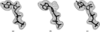Figure 1 (a) ATP fitted into model 2.5 Å density for ATP. (b) ddATP fitted into model density for ATP. (c) GTP fitted into model density for ATP.

#### 3.2. Identification of ligands based on correlation of densities after fitting

A simple approach to identification of a ligand from experimental (FoFc)exp(iφc) electron density would be to fit a set of candidate ligands to this density, scoring each based on the correlation of (fitted) model density to the experimental density in the region of the model and choosing the highest scoring ligands as the most likely to be correct. We tested this procedure using the set of 119 unique ligands selected above (obtained by clustering the most common 200 ligands from the PDB at a threshold correlation of 0.85).

For each ligand, a PDB entry containing the ligand was chosen as described in §2, the ligand was removed from the entry and (FoFc)exp(iφc) difference density was calculated. An example of each of the 119 unique ligands (from a different PDB entry if possible, as described in §2) was then fitted into this difference density and the correlation of resulting model density and observed difference density was calculated.

Fig. 2(a) shows the utility of the correlation coefficient in identifying ligands based on difference density. For the set of 119 unique ligands, the rank number of the correct ligand (i.e. that in the PDB entry from which the density was obtained) is shown. Overall, in 46% of cases the ligand with the highest correlation was the correct ligand. In most remaining cases the correct ligand was within the top-ranked few ligands, but some were as low in rank as number 14.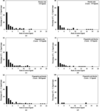Figure 2 Histograms of rank position of correct ligands. (a) Scoring using correlation of density, considering 119 unique ligands. (b) Scoring using Z score derived from correlation of density. (c) Scoring using Z score derived from correlation of fingerprints of density and fingerprints of model density. (d) Scoring using sum of Z scores from correlation of density and correlation of fingerprints of density. (e) As in (d), but considering all 200 of the most common ligands in the PDB. (f) As in (d), but considering only 31 unique ligands.

The reason why some of the ligands could be identified with this approach and others could not is likely to be that some density is relatively unique in shape, allowing substantial discrimination among ligands, while other density is not and many ligands can fit into it. Fig. 3illustrates examples of ligand density that could be fitted well by only one ligand among the 200 most common from the PDB. Difference density for bacteriochlorophyll a at a resolution of 2.35 Å (PDB code 1ogv ; Katona et al., 2003), for example, is highly distinctive, as is density for cyclohexyl-hexyl-β-D-maltoside at a resolution of 1.1 Å (PDB code 1ong ; Nukaga et al., 2003).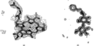Figure 3 (a) Fo − Fc difference density for bacteriochlorophyll a at 2.4 Å (PDB code 1ogv ; Katona et al., 2003), fitted with the same ligand from PDB entry 1dv6 (Axelrod et al., 2000). (b) Difference density for cyclohexyl-hexyl-β-D-maltoside at a resolution of 1.1 Å (PDB code 1ong ; Venkatesan et al., 2004), fitted with the same ligand from PDB entry 1q2p (Nukaga et al., 2003).

Fig. 4illustrates an example of density that can be fitted by many ligands. The (FoFc)exp(iφc) difference density for tris-(hydroxylmethyl)-aminomethane is from PDB entry 1m6z (A. Noergaard, P. Harris, S. Larsen & H. E. M. Christensen, unpublished work) at a resolution of 1.4 Å. It can be fitted by this same ligand (Fig. 4a) with a correlation of 0.72, but it can also be fitted even better by several other ligands such as oxalate (Fig. 4b, correlation of 0.76) or dioxane (Fig. 4c, correlation of 0.76).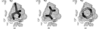Figure 4 Fitting of Fo − Fc difference density for tris-(hydroxylmethyl)-methane from PDB entry 1m6z (A. Noergaard, P. Harris, S. Larsen & H. E. M. Christensen, unpublished) at a resolution of 1.4 Å. (a) Density fitted by the same ligand from a different PDB entry (1s18 ; Dai et al., 2004). (b) Density fitted with oxalate. (c) Density fitted with dioxane.

#### 3.3. Identification of ligands based on Z scores using correlation of densities after fitting

Some density can be readily fitted by several ligands as shown above and conversely some ligands can fit most density better than other ligands. For example, the mean ± SD of correlation of density after fitting tris-(hydroxylmethyl)-aminomethane to all 119 unique observed ligand difference density maps was 0.61 ± 0.08, while the same quantities for dioxane were 0.68 ± 0.08. Therefore, it might be reasonable to conclude that a fit of tris-(hydroxylmethyl)-methane that had a correlation of 0.61 is approximately equivalent to a fit of dioxane with a correlation of 0.68. We used a Z-score approach to carry out this normalization, with the Z score given by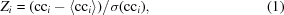where cci is the correlation of model density for ligand i to the FoFc difference density after fitting and 〈cci〉 and σ(cci) are the mean and SD of correlations of ligand i to all 119 difference density maps. In essence, 〈cci〉 and σ(cci) are the mean and SD of the correlation of ligand i to representative difference density from the PDB.

Fig. 2(b) shows the use of Z scores based on correlation coefficient in identifying ligands. The Z-score normalization increases the percentage of cases where the ligand with the highest correlation was the correct ligand from 46% to 64%.

#### 3.4. Identification of ligands based on fingerprints of correlation coefficients

The process of fitting each of 119 ligands to difference density and obtaining correlation coefficients for each fit yields some information that we have not taken full advantage of by simply choosing the highest correlation or Z score to identify the best-fitting ligand. This additional information is the pattern of fits of the entire set of 119 ligands. Fig. 5illustrates the fingerprints for difference density for tris-(hydroxylmethyl)-methane and for ATP. The correlation coefficients for each of the 119 ligands are shown, where the ligands are sorted on the basis of the number of non-H atoms. The fingerprint for tris-(hydroxylmethyl)-methane shows that many small ligands fit well to its difference density, while large ligands do not. In the case of ATP, the pattern is much more complicated, with some small and some large ligands fitting well and others not.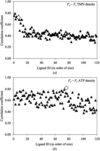Figure 5 Fingerprints of difference density. (a) Correlation of each of 119 unique ligands after fitting to difference density for tris-(hydroxyamino)-methane from PDB entry 1m6z (A. Noergaard, P. Harris, S. Larsen & H. E. M. Christensen, unpublished work) at a resolution of 1.4 Å. The ligands are sorted from left to right based on increasing numbers of non-H atoms. (b) As in (a), except fitting to difference density for ATP from PDB entry 1aq2 at a resolution of 1.9 Å (Tari et al., 1997). The correlations are all indicated by filled triangles, except for the correlation of the correct ligand, which is indicated by an open diamond.

We use the correlation of the single fingerprint calculated from the difference density to be identified, with the fingerprints obtained for model density for each of the 119 ligands considered as a second measure of the compatibility of the density with each of those 119 ligands. We calculate this as a Z score in the same fashion as described above for single correlation coefficients.

Fig. 2(c) shows the use of Z scores based on correlation of fingerprints derived from difference density with fingerprints for each ligand using model data. This approach (without including any Z-score information directly on the fit of the individual ligand to the density) is capable of identifying 41% of the 119 ligands (Fig. 2c). When combined by simple summation with the Z score based on correlation coefficient, 68% of the top-ranked ligands are the correct ligands (Fig. 2d).

We examined how the accuracy of identification varies with the number of possible ligands considered. Fig. 2(e) shows that if all 200 of the most common ligands are considered, then 48% of the top-ranked ligands are correct. If only 31 ligands are considered (Fig. 2f), 90% of the top-ranked ligands are correct.

### 4. Conclusions

We find that our combination of two measures of the characteristics of ligand density, a Z score based on the correlation of the density with model density from fitted ligands and a Z score based on the correlation of the fingerprint of the density with model fingerprints of the same ligands, can be of considerable utility in identifying the correct ligand. The summed Z scores Zi that are used can be converted to approximate estimates of relative probabilities with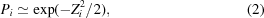allowing a probabilistic assessment of the ranking of ligands that may correspond to the experimental density. This in turn allows the construction, for example, of a list of all the ligands with probability greater than 0.2 or a list of the ligands that, considered together, make up a cumulative probability of 0.5. If there are only a few possible ligands to consider and these ligands are dissimilar in shape, then this approach can reliably identify which ligand is present, as in Fig. 2(f). If there are many ligands, then the identification will consist more often of a group of ligands that are similar to each other, any of which might be the ligand present in the crystal structure.

There are a number of improvements that might be made to this method. Probably the most important one will be to include the contacts between ligand and macromolecule and other compounds present in the crystal in the scoring of the fit of the ligands. Many of the alternatives for ligand placement are likely to form implausible contacts, allowing them to be eliminated or at least reduced in probability. Other improvements might include resolution-dependent and possibly noise-dependent tables of correlations of model ligands and ligand density and the use of difference maps after fitting to evaluate the quality of fit of a ligand to density.

### Acknowledgements

The authors would like to thank Li-Wei Hung, Eleanor Dodson and Tom Peat for discussion and the NIH (P01GM063210) for generous support of the PHENIX project.

### ReferencesBIOLOGICALCRYSTALLOGRAPHY